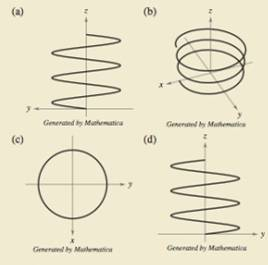Chapter 12.1, Problem 82E

Chapter
Section
Textbook Problem

# HOW DO YOU SEE IT? The four figures below are graphs of the vector-valued function r ( t ) = 4 cos t i + 4 sin t j + t 4 k Match each of the four graphs with the point in space from which the helix is viewed.(i) (0, 0, 20)(ii) (20, 0, 0)(iii) (-20, 0, 0)(iv) (10, 20, 10)

Problem A:

To determine

To Calculate: Match each of the four graphs with the points in space which the helix is viewed.

Explanation

Given: r(t)=4costi+4sintj+t4<

Problem b:

To determine

To Calculate: Match each of the four graphs with the points in space which the helix is viewed.

Problem c:

To determine
Match each of the four graphs with the points in space which the helix is viewed.

Problem d:

To determine

To Calculate: Match each of the four graphs with the points in space which the helix is viewed.

### Still sussing out bartleby?

Check out a sample textbook solution.

See a sample solution

#### The Solution to Your Study Problems

Bartleby provides explanations to thousands of textbook problems written by our experts, many with advanced degrees!

Get Started